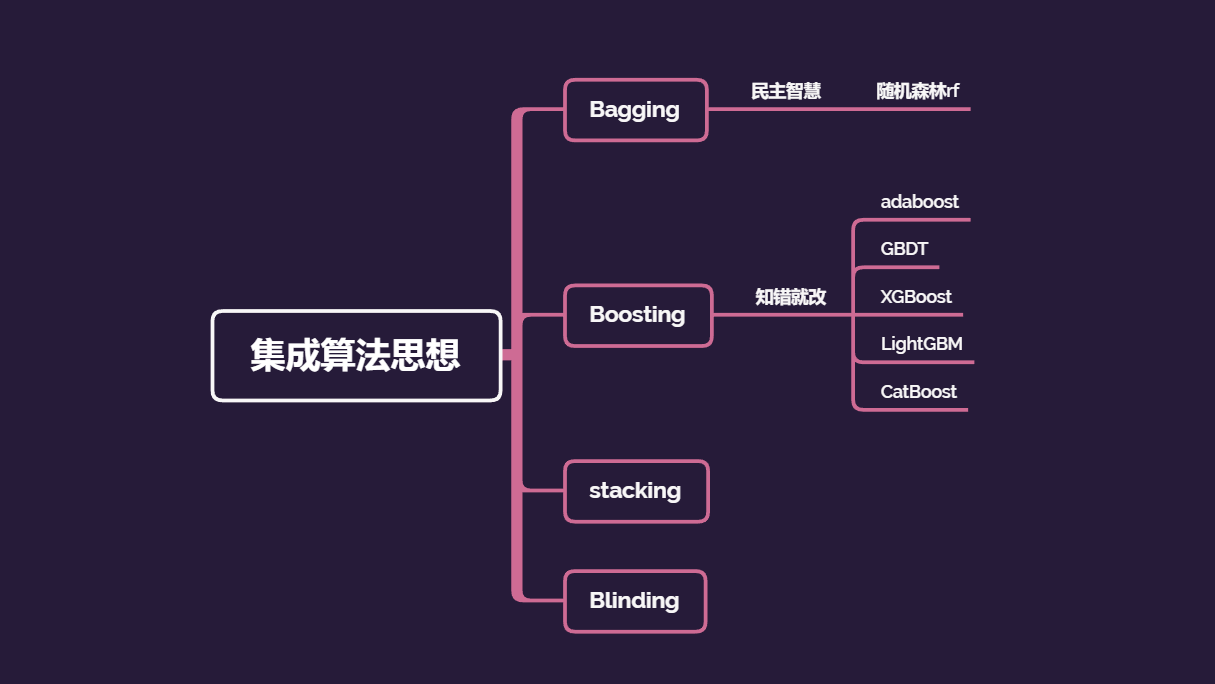# 【python 机器学习】机器学习算法之CatBoost

2017年俄罗斯的搜索巨头 Yandex 开源 Catboost 框架。Catboost（Categorical Features+Gradient Boosting）采用的策略在降低过拟合的同时保证所有数据集都可用于学习。性能卓越、鲁棒性与通用性更好、易于使用而且更实用。据其介绍 Catboost 的性能可以匹敌任何先进的机器学习算法。CatBoost这个名字来自两个词“Category”和“Boosting”。如前所述，该库可以很好地处理各种类别型数据，是一种能够很好地处理类别型特征的梯度提升算法库。

CatBoost的安装非常的简单，只需执行pip install catboost即可。

# -*- coding: utf-8 -*-
from sklearn.metrics import *
from sklearn.model_selection import train_test_split
import pandas as pd

from catboost import CatBoostRegressor

# 读取数据
data_path='train_data.txt'
# 导入数据

# 筛选自变量
X=data.iloc[:,1:]
# 筛选因变量
y=data.iloc[:,0]
# 提取特征名
feature_names=list(X.columns)
# 切分数据，划分为训练集和测试集
X_train, X_test, y_train, y_test = train_test_split(X, y, test_size=0.3, random_state=33)

params = {
'iterations':330,
'learning_rate':0.1,
'depth':10,
'loss_function':'RMSE'

}

clf = CatBoostRegressor(**params)
clf.fit(X_train, y_train,verbose=3)

# 效果评估，均方误差,均方根误差，R2
y_predict=clf.predict(X_test)
mae=mean_absolute_error(y_test, y_predict)
mse =mean_squared_error(y_test, y_predict)
rmse=round(mse **0.5,4)
r2=round(r2_score(y_test, y_predict),4)

# 评估指标
print("MAE: %.4f" % mae)
print("MSE: %.4f" % mse)
print("RMSE: %.4f" % rmse)
print("R2: %.4f "% r2 )



cv_params  = {'depth': [8,9,10,11,12,13,14]}

other_params = {
'iterations': 800,
'learning_rate': 0.09,
# 'depth': 10,
'loss_function': 'RMSE'
}

cat_model_ = CatBoostRegressor(**other_params)
cat_search = GridSearchCV(cat_model_,
param_grid=cv_params ,
scoring='neg_mean_squared_error',
iid=False,n_jobs=-1,
cv=5)

cat_search.fit(X_train, y_train)

means = cat_search.cv_results_['mean_test_score']
params = cat_search.cv_results_['params']

print(means)
print(params)
print(cat_search.best_params_)
print(cat_search.best_score_)


CatBoost参数详解

loss_function 损失函数，支持的有RMSE, Logloss, MAE, CrossEntropy, Quantile, LogLinQuantile, Multiclass, MultiClassOneVsAll, MAPE, Poisson。默认RMSE。
custom_metric 训练过程中输出的度量值。这些功能未经优化，仅出于信息目的显示。默认None。
eval_metric 用于过拟合检验（设置True）和最佳模型选择（设置True）的loss function，用于优化。
iterations 最大树数。默认1000。
learning_rate 学习率。默认03。
random_seed 训练时候的随机种子
l2_leaf_reg L2正则参数。默认3
bootstrap_type 定义权重计算逻辑，可选参数：Poisson (supported for GPU only)/Bayesian/Bernoulli/No，默认为Bayesian
bagging_temperature 贝叶斯套袋控制强度，区间[0, 1]。默认1。
subsample 设置样本率，当bootstrap_type为Poisson或Bernoulli时使用，默认66
sampling_frequency 设置创建树时的采样频率，可选值PerTree/PerTreeLevel，默认为PerTreeLevel
random_strength 分数标准差乘数。默认1。
use_best_model 设置此参数时，需要提供测试数据，树的个数通过训练参数和优化loss function获得。默认False。
best_model_min_trees 最佳模型应该具有的树的最小数目。
depth 树深，最大16，建议在1到10之间。默认6。
ignored_features 忽略数据集中的某些特征。默认None。
one_hot_max_size 如果feature包含的不同值的数目超过了指定值，将feature转化为float。默认False
has_time 在将categorical features转化为numerical features和选择树结构时，顺序选择输入数据。默认False（随机）
rsm 随机子空间（Random subspace method）。默认1。
nan_mode 处理输入数据中缺失值的方法，包括Forbidden(禁止存在缺失)，Min(用最小值补)，Max(用最大值补)。默认Min。
fold_permutation_block_size 数据集中的对象在随机排列之前按块分组。此参数定义块的大小。值越小，训练越慢。较大的值可能导致质量下降。
leaf_estimation_iterations 计算叶子值时梯度步数。
leaf_estimation_backtracking 在梯度下降期间要使用的回溯类型。
fold_len_multiplier folds长度系数。设置大于1的参数，在参数较小时获得最佳结果。默认2。
approx_on_full_history 计算近似值，False：使用1／fold_len_multiplier计算；True：使用fold中前面所有行计算。默认False。
class_weights 类别的权重。默认None。
scale_pos_weight 二进制分类中class 1的权重。该值用作class 1中对象权重的乘数。
boosting_type 增压方案
allow_const_label 使用它为所有对象训练具有相同标签值的数据集的模型。默认为False
CatBoost默认参数：

‘iterations’: 1000,
‘learning_rate’:0.03,
‘l2_leaf_reg’:3,
‘bagging_temperature’:1,
‘subsample’:0.66,
‘random_strength’:1,
‘depth’:6,
‘rsm’:1,
‘one_hot_max_size’:2
‘fold_len_multiplier’:2,
‘border_count’:128,
CatBoost参数取值范围：

‘learning_rate’:Log-uniform distribution [e^{-7}, 1]
‘random_strength’:Discrete uniform distribution over a set {1, 20}
‘one_hot_max_size’:Discrete uniform distribution over a set {0, 25}
‘l2_leaf_reg’:Log-uniform distribution [1, 10]
‘bagging_temperature’:Uniform [0, 1]
‘gradient_iterations’:Discrete uniform distribution over a set {1, 10}‘


©️2019 CSDN 皮肤主题: 编程工作室 设计师: CSDN官方博客